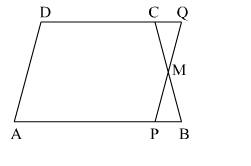# In a trapezium ABCD, AB || DC and M is the midpoint of BC.

Question:

In a trapezium ABCDAB || DC and is the midpoint of BC. Through M, a line PQ || AD has been drawn which meets AB in P and DC produced in Q, as shown in the adjoining figure. Prove that ar(ABCD) = ar(APQD).Solution:

In MQC and MPB,
MC = MB                            (M is the midpoint of BC)
CMQ = BMP                (Vertically opposite angles)
MCQ = MBP                (Alternate interior angles on the parallel lines AB and DQ)
Thus, MQC  MPB   (ASA congruency)
ar(MQC) = ar(MPB)
ar(MQC) + ar(APMCD) = ar(MPB) + ar(APMCD)
ar(APQD) = ar(ABCD)